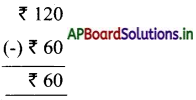# AP Board 5th Class Maths Solutions 3rd Lesson Addition and Subtraction

Andhra Pradesh AP Board 5th Class Maths Solutions 3rd Lesson Addition and Subtraction World Textbook Exercise Questions and Answers.

## AP State Syllabus 5th Class Maths Solutions Chapter 3 Addition and Subtraction

Shankar cultivated paddy in his two acres field. He wanted to know the cost of cultivation. He asked his daughter to write down the details of his expenditure. She wrote the details as follows.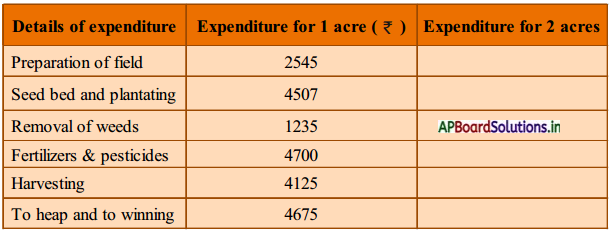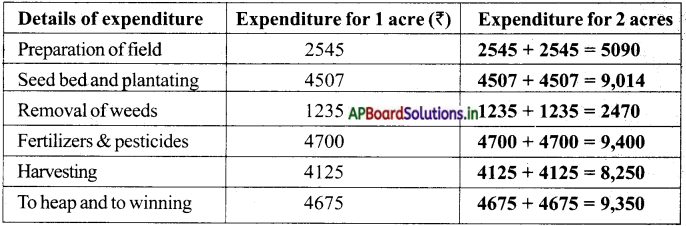Question 1.
How much did Shankar invest on preparation of field for seed bed?
Investment for field prepantion = 2545
Investment for seed bed = 4507
Total investment = 7052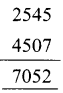∴ For 2 acres = 7052 + 7052 = 14104.Question 2.
How much did he spend on harvesting and to heap and winning?
Investment spent on harvesting = 4125
Investment spent on heapand winning = 4675
Total investment = ₹ 8800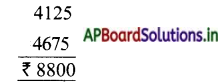∴ For 2 acres 8800 + 8800 = 17600.

Question 3.
How much did he spend on seed bed and removal of weeds ?
Investment spent on seed bed = ₹ 4507
Investment spent on removal of weeds = ₹ 1235
Total investment = ₹ 5742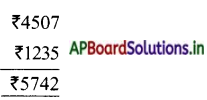∴ For 2acres = 5742 + 5742 = ₹ 1484

Question 4.
What is the total cost of cultivation of paddy in 1 acre ?
Total cost of cultivation of paddy in 1 acre = ₹ 21,787

Question 5.
What is the total cost of cultivation of paddy in 2 acres ?
Sambaiah, the brother of Shankar, has 1 acre of field.
Total cost of cultivation of paddy in 2 acre = ₹ 43,574Do these : (TextBook Page No.33)

I. Do the following: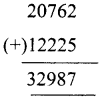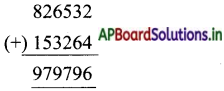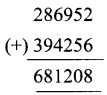II. Arrange in columns and add:

1) 932648 + 643578 + 376493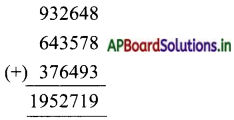2) 763482 + 367842 + 567324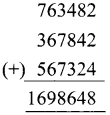3) 673483 + 447862 + 663822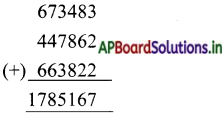4) 25014 + 203101 + 2020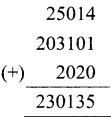Mohan is proprietor of a meals canteen. He spent 31,787/- in January – 2020 and earned 53,574/-. Calculate his gain in the month.
Mohan earned in January: 53,574/-
He spent: 31,787/-
gain in the month = 21,787/-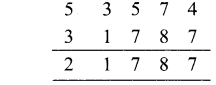Do these: (TextBook Page No.34)

Question 1.
95,579

b. 56080 – 4398
51,682

c. 600005 – 65095
5,34,910

d. 880056 – 45396
8,34,660

e. 700000 – 75897
6,24,103

f 906004 – 473894
4,32,110

g. 435217 – 383450
51,767

h. 980000 – 573429
4,06,571

i. 650701 – 404107
2,46,504.Question 2.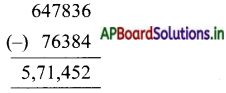b. Subtract 8437 from 783409.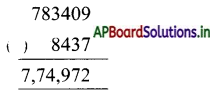c. Subtract 386472 from 764986.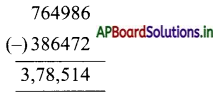d. Subtract 432010 from 705645.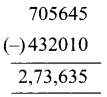e. Subtract 607080 from 900000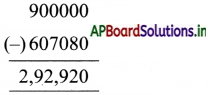f. Subtract 201781 from 400000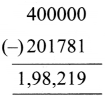Question 3.
Difference between 503267 to 153672 is 349,595
∴ 3,49,595 is to be added to 153672 to get 503267.

b. What is to be added to 603257 to get 999999
Difference between 999999 to 603257 is 396,742
∴ 3,96,742 is to be added to 603257 to get 999999.

c. By how much 20325 is less than 425067 ?
Difference between 425067 and 20325 is 404,742
∴ 20325 is 4,04,742 less than 425067

d. How much is to be subtracted from 673267 to make it to 59325 ?
Difference between 673267 to 59325 is 6,13,942
∴ 6,13,942 is be subtracted from 673267 to make it to 59325.Cloth Store: (TextBook Page No.35)

Vanaja’s family decided to purchase some garments on the occasions of her daughter’s marriage. So she pur¬chased some garments in APCo showroom. The billing details are as shown below.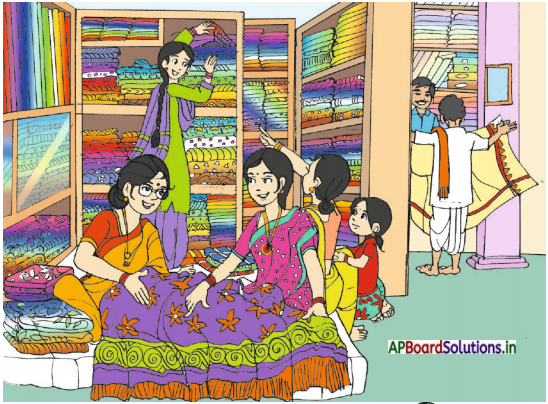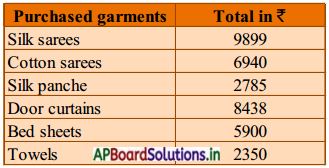Question 1.
How much money is spent on silk sarees more than that of door curtains?
Money spent on silk sarees is = 9899
Money spent on door curtains is = 8438
Difference = 1,461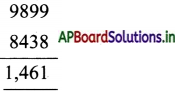₹ 1,461 is more than silk sarees than that of door curtains.Question 2.
How much less money is spent on bed sheets than cotton sarees ?
Money spent on cotton sarees= 6940
Money spent on Bed sheets = 5900
Difference = 1040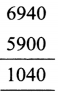₹ 1,040 is spent less than on bed sheets than that of cotton sarees.

Question 3.
How much money is spent on both silk sarees and silk panche ?
Money spent on silk sarees = 9899
Money spent on silk panche = 2785
Total money spent = 12,684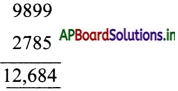Question 4.
How much money did Vanaja spend for door curtains, bed sheets and towels ?
Money spent on door curtains = 8438
Money spent on bed sheets = 5900
Money spent on towels = 2350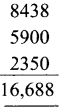Total spent amount = 16,688.Question 5.
How much money did Vanaja spend for shopping ?
Money spent for shopping by Vanaja = 9899 + 6940 + 2785 + 8438 + 5900 + 2350 = ₹ 36,312 .

Exercise 1:

I. Do the following.
Answer: 4986 + 3430 – 5467 = ______
2949

b. 78645 – 36789 + 23576 = ______
65,432

c. 40376 – 20568 – 76485 + 87364 = ______
30,687

d. 643857 + 467896 – 445386 = ______
666,367

Question 2.
Fill in the blanks.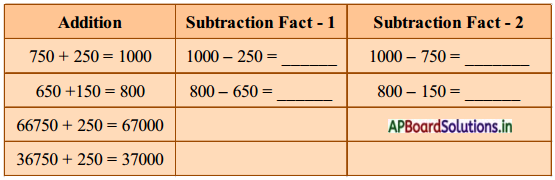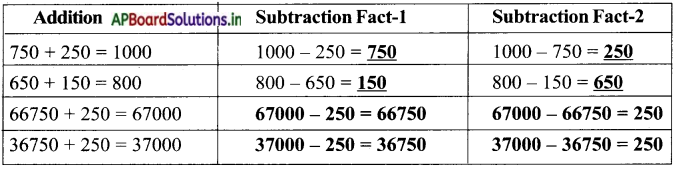Question 3.
A man earns ₹ 37645 in a month and his wife earns ₹ 25367. If they spend ₹ 38600 in a month, how much do they save in that month?
Earning of a man in a month = ₹ 37645
Earning of his a wife in a month = ₹ 25367
Total earnings in a month = ₹ 63012
Total Spending amount in a month = ₹ 38600
Saving amount in a month = ₹ 24,412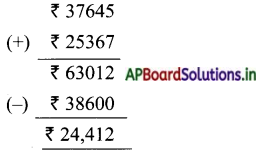Question 4.
Siva had ₹ 52,490. He purchased a cow for ₹ 15,870 and a buffalo for ₹ 25,785. How much money is with him now?
Costofacow = ₹ 15,870
Cost of Buffalo = ₹ 25,785
Total spending amount = ₹ 41,655
Amount at Siva = ₹ 52,490
Remaining amount = ₹ 52,490 – 41,655 = ₹ 10,845

Question 5.
A milk dairy produces 25,545 litres of milk every day. It supplies 15,625 litres of milk to various milk depots and the rest to the market. How much milk is supplied to the market?
Production of millc in dairy on every day = 25,545
Supply of milk to milk depots = (-) 15,625
Supply of milk to market = 9,920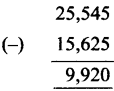Do this: (TextBook Page No.78)

Question 1.
Fill in the blanks using addition properitles.

1. 35 + 67 = 67 + ____
35

2. 378 + 894 = ____ + ____
894 + 378

3. 889 + 0 = ________
0 + 889

4. 0 + ____ = 6592 + 0
6592

5. 7634 + 3110 = 3210 + 7634
3210

6. 9345 + ____ = 4537 + ____
4537, 9345Exercise 2:

Workout the Problems:

Question 1.
Cost of pesticide sprayer is ₹ 4500/- Government provides a subsidy of ₹ 2900/- How much farmer has to pay from his pocket?
Cost of pesticide sprayer = ₹ 4500
Subsidy amount on sprayer = ₹ 2900
Money has to pay by the farmer = ₹ 1600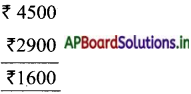Question 2.
What is the difference of largest 5 digit number and smallest 6 digit number?
Smallest 6-digit number = 100,000
Greatest 5-digit number = 99,999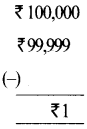Difference = ₹ 1.

Question 3.
A man earned ₹ 4,75,000 in a year. He spent ₹ 3,85,600. How much money did he save?
Earnings ofa man in a year = ₹ 4,75,000
Spendings ofa man in a year = ₹ 3,85,600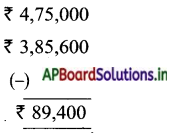Savings of a man in a year = ₹ 89,400Question 4.
There are 3,25,208 men; 3,18,405 women and 2,98,405 children in a town. What is the total population of the town ?
Men in a town = 3,25,208
Women in a town = 3,18,405
Children in a town = 2,98,405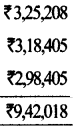Total population in the town = 9,42,018

Question 5.
In a district 36,405 students were passed in S.S.C examinations. If the number of failed students were 4,305 find the total number of students appeared for the examination.
Number of students who passed in the examination = 36,405
Number of students who failed in the examination = 4,305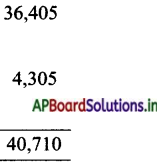Number of students who appeared in the examination = 40,710

Question 6.
Padmaja’s income was ₹ 5,35,256 in 2018. Next year her income exceeded the previous year’s income by ₹ 78,500. What was her income in the year 2019? How much did she earn in these two years ?
Next year her income exceeded = ₹ 78,500
Padmaja’s income in 2019 = ₹ 5,35,256 + ₹ 78,500 = ₹ 6,13,756
Total income in two years = ₹ 5,35,256 (+) ₹ 6,13,750 = ₹ 11,49,012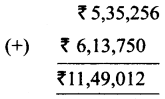TextBook Page No.41:

Question 1.
Raghu went to Rythu bazar and bought some vegetables worth ₹ 158/- grocery worth ₹ 143/-. How much he spent approximately ?
a. ₹ 200
b. ₹ 300
c. ₹ 400
d. ₹ 500
b. ₹ 300

Question 2.
Raju bought a mobile for ₹ 7890/- and a chair for ₹ 3295/- . Estimate how much did he pay more for the mobile.
a. ₹ 4000
b. ₹ 3000
c. ₹ 1000
d. ₹ 5000
d. ₹ 5000

Question 3.
Haseena bought a saree for ₹ 5345/- and a shirt for ₹ 2050/-. Estimate the amount she has to pay to the shop keeper approximately.
a. ₹ 5000
b. ₹ 4000
c. ₹ 7000
d. ₹ 2000
c. ₹ 7000

Question 4.
Bunny scored 6,776 points on a video game and Baba scored 2,373 points. Estimate the difference of the scores of Bunny and Baba approximately.
a. 5000
b. 8000
c. 7000
d. 6000
a. 5000

Question 5.
Lakshmi is reading a book that contain 257 pages. She has already read 163 pages. Estimate how many pages are yet to be read approximately ?
a. 600
b. 900
c. 100
d. 70
c. 100

Profit and Loss (TextBook Page No.41):

A weaver weaves sarees on his handlooms and sells them in markeL He buys cotton, thread, silk thread, jery silk … etc, from a whole-seller. He sells them at a profit and sometimes at a loss. He weaves costly sarees on the orders of consumers. He can weave a cotton-saree within two days and a silk saree in 4 to 5 days. Now let’s see his cost of measuring each saree.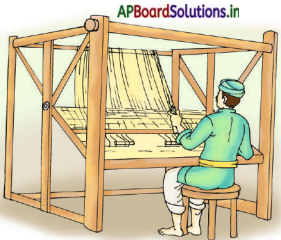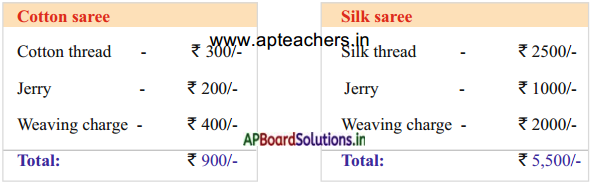Question 1.
Weaver sells cotton saree for ₹ 1100/ – Does he make a profit or a loss ?
Selling price > Cost price.
So he make a profit.

Question 2.
Weaver sells damaged cotton saree for ₹ 400/- each. Does he make a profit or a loss ?
Selling price < Cost price.
So he make a loss.

Question 3.
Weaver sells silk sarees for ₹ 6000/- does he make a profit or a loss ?
Selling price > Cost price.
So he make a profit.

Exercise 3:

Find the amount of profit or loss for the following problems.

Question 1.
Cost price of rice bag = ₹ 750 ; Selling price = ₹ 900
Cost price of rice bag = ₹ 750
Selling price of rice bag = ₹ 900
S.P > C.P. So profit is possible.
∴ Profit = S.P – C.P = 900 – 750 = ₹ 150.

Question 2.
Cost price of bed sheet = ₹ 635 ; Selling price = ₹ 815
Cost price of bedsheeet = ₹ 635
Selling price of bedsheeet = ₹ 815
S.P > C.P. So profit is possible.
∴ Profit = S.P – C.P = 815 – 635 = ₹ 180

Question 3.
Cost price of umbrella = ₹ 105; Selling price = ₹ 90
Cost price of umbrella = ₹ 105
Selling price of umbrella = ₹ 90
S.P < C.P. So Loss is possible.
Loss = C.P – S.P = 105 – 90 = ₹ 15

Question 4.
Cost price of a fan is ₹ 800 and by selling it, Ravi got a profit of ₹ 250. What is its selling price ?
Cost price of fan = ₹ 800
Selling price of fan = ₹ 250
Selling price of a fan = C.P + Profit = 800 + 250 = ₹ 1050.

Question 5.
Cost price of a motor cycle is jaygot a loss of ₹ 1800. What is its selling price ?
Cost price of a motor cycle = ₹ 42,500
Gain loss = ₹ 1800
Selling price of motor cycle = C.P – loss = ₹ 40,700

Question 6.
A thermos flask is purchased for ₹ 450 by a shopkeeper. He wants a profit of ?50. What should be its selling price ?
Required profit = ₹ 50
Selling price of a flask = ₹ 500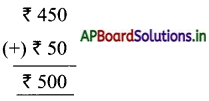Question 7.
Rekha and Geetha went to a movie. Rekha bought two tickets for ₹ 120. Geetha bought two pop-corn packets at the rate of ₹ 30 for each. How much money did Rekha spend more than that of Geetha ?